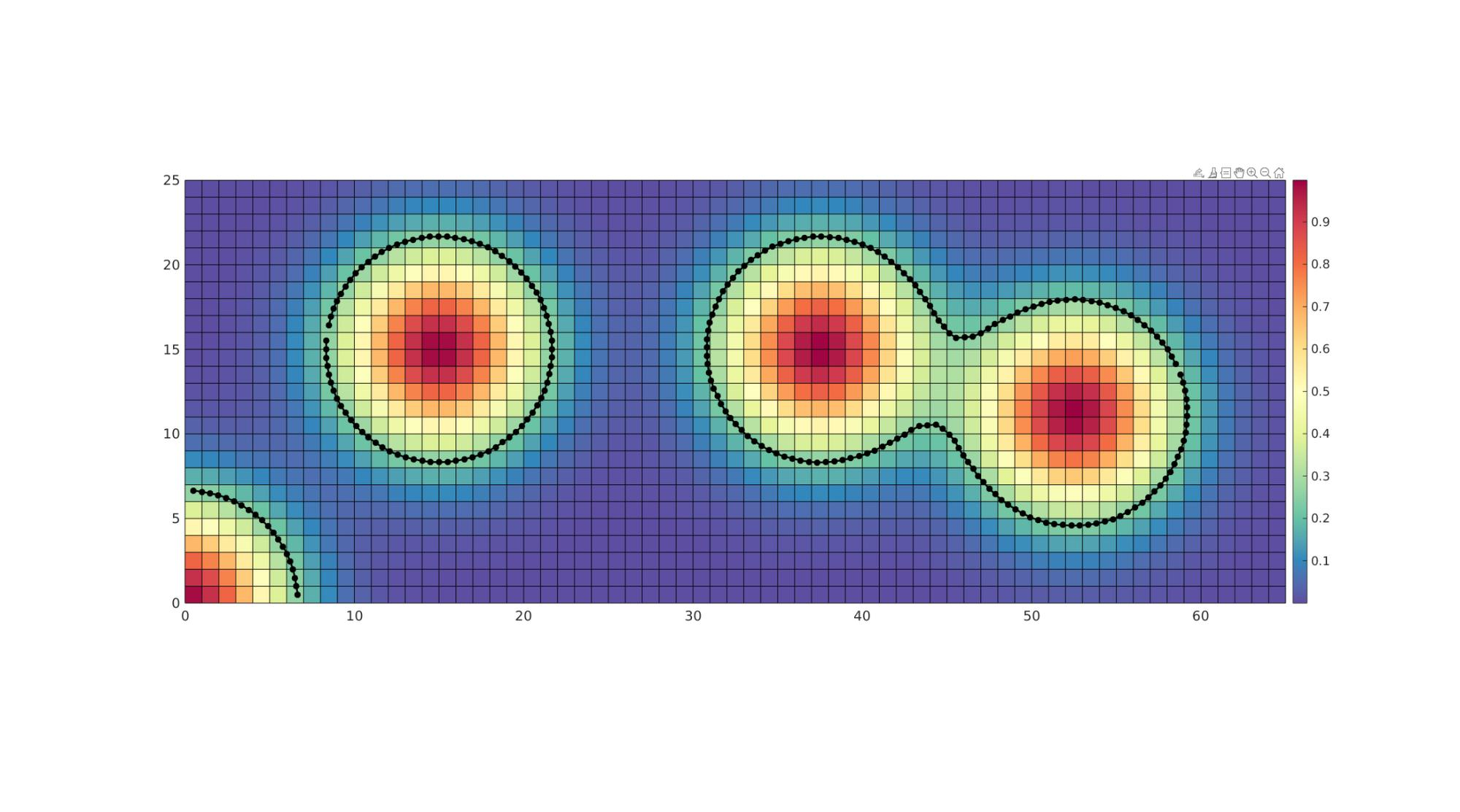# gcontour

Below is a demonstration of the features of the gcontour function

## Contents

```clear; close all; clc;
```

## Syntax

[C,cSiz,cLevel]=gcontour(X,Y,Z,k,pointSpacing,resampleMethod);

## Description

Computes contours for the image with pixel centre coordinates X and Y and intensity Z, at the intensity level k. Contours are resampled using a point spacing equal to pointSpacing using resampleMethod as the interpolation method.

## Examples

Plot settings

```lineWidth=2;
markerSize=25;
fontSize=15;
```

## Example 1: Obtainin a single contour

```S=4;

v=[1 1 1];
siz=[25 65];
[J,I]=meshgrid(1:1:siz(2),1:1:siz(1));
K=zeros(size(I));
[X,Y,Z]=im2cart(I,J,K);

xc=15;
yc=15;
M=exp(-((X-xc).^2 + (Y-yc).^2)./(2*S^2)) + ...
exp(-((X-2.5*xc).^2 + (Y-yc).^2)./(2*S^2)) + ...
exp(-((X-3.5*xc).^2 + (Y-0.75*yc).^2)./(2*S^2)) + ...
exp(-((X).^2 + (Y).^2)./(2*S^2));

contourLevel=0.25;
pointSpacing=0.5;
resampleMethod='pchip';

%Compute contour
VC=gcontour(X,Y,M,contourLevel,pointSpacing,resampleMethod);

[Fm,Vm,Cm]=im2patch(M,true(size(M)),'sk',v);
Vm(:,3)=0;
```

Visualization

```cFigure; hold on;
gpatch(Fm,Vm,Cm);
for q=1:1:numel(VC)
plotV(VC{q},'k.-','LineWidth',lineWidth,'MarkerSize',markerSize)
end
colormap spectral; colorbar;
axis tight; axis equal; box on;
set(gca,'FontSize',fontSize)
drawnow;
```## ExamplesGIBBON www.gibboncode.org

Kevin Mattheus Moerman, [email protected]

GIBBON footer text

GIBBON: The Geometry and Image-based Bioengineering add-On. A toolbox for image segmentation, image-based modeling, meshing, and finite element analysis.

Copyright (C) 2006-2023 Kevin Mattheus Moerman and the GIBBON contributors

This program is free software: you can redistribute it and/or modify it under the terms of the GNU General Public License as published by the Free Software Foundation, either version 3 of the License, or (at your option) any later version.

This program is distributed in the hope that it will be useful, but WITHOUT ANY WARRANTY; without even the implied warranty of MERCHANTABILITY or FITNESS FOR A PARTICULAR PURPOSE. See the GNU General Public License for more details.

You should have received a copy of the GNU General Public License along with this program. If not, see http://www.gnu.org/licenses/.